# 2021-ICLR-An Image is Worth 16x16 Words：Transformers for Image Recognition at Scale

## 2. 引言

• Wang et al. 2018就提出，可以先使用一个 CNN 来提取图像的特征，然后将特征展开作为图像的序列数据。比如 ResNet50，最后提取得到的特征 Map 只有 $14 \times 14$，展开成一维就得到 $196$ 长度的序列数据。

1. locality：即图片上相邻的区域会有相邻的特征；
2. translation equivariance：即对图片先做平移后做卷积，和先做卷积后做平移，最后的结果都是一样的，即平移等变性。

## 3. 架构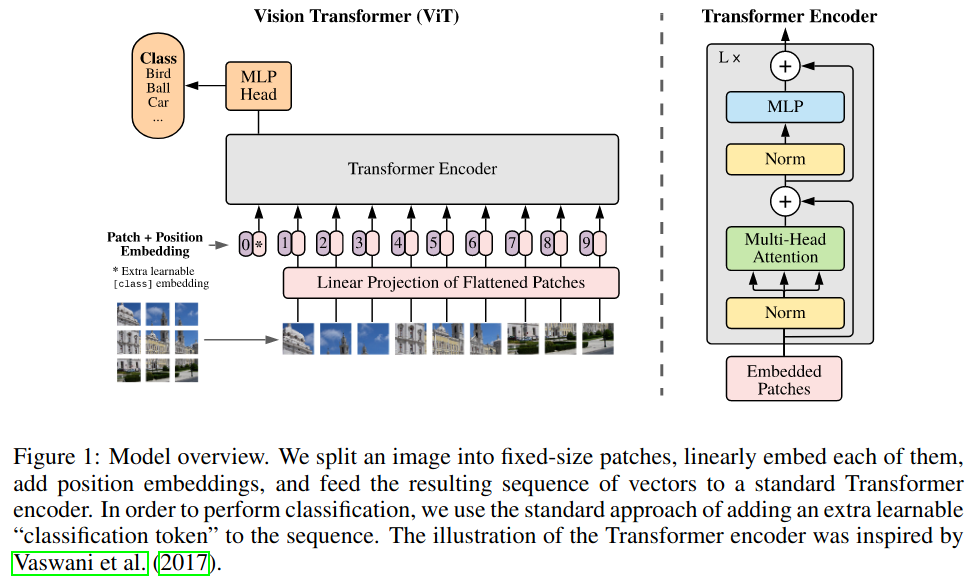ViT 的整体流程：首先先将图片划分为许多 $16 \times 16$ 的 patch，然后经过一个线性投射层得到每个 patch 的编码向量，再将位置编码加入编码向量，同时引入一个全局 patch $*$ 及其位置编码（即类似 BERT 中的 [cls] token），一同输入 Transformer 编码器中，最后只取 patch $*$ 对应的输出，过一个 MLP Head 后用于分类，使用交叉熵损失。从上图右边可以看到，ViT 使用的就是标准的 Transformer 编码器。具体来说，如果图片大小为 $224 \times 224$，则

• patch 个数：$(224 / 16)^2 = 196$;
• patch 大小：$16 \times 16 \times 3 = 768$
• 输入向量：$196 \times 768$
• 线性投射层输出：$196 \times 768$
• 加上位置编码向量：$196 \times 768$（位置编码向量长度为 $768$）；
• Transformer 编码器输入：$197 \times 768$（加上了 patch $*$）；

## 4. 消融

### 4.1 [cls] token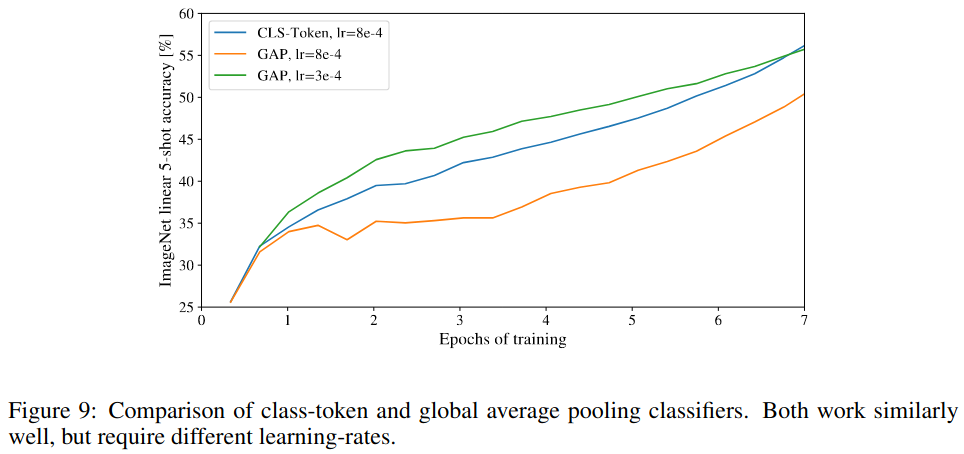### 4.2 位置编码

• $1$-D 位置编码：对图像 patch 使用光栅扫描展成一维序列；
• $2$-D 位置编码：将图像 patch 看作是栅格上的一点，如果位置编码的长度为 $D$，就分别使用 $\frac{D}{2}$ 的向量来表示每个栅格点的横纵坐标，最后再拼接得到 $D$ 长的位置编码；
• 相对位置编码：在进行 Transformer 编码器前，做一次额外的注意力：对 Query $p_q$ 和 Key $p_k$ 使用使用相对注意力，即用 $p_q - p_k$ 表示这两个 patch 的相对位置信息，然后对 $p_q - p_k$ 做 Embedding 后再计算注意力，这样就将相对位置信息加入到了输入数据中。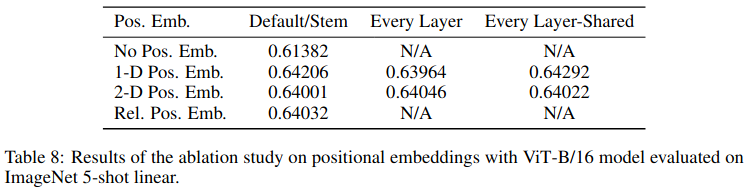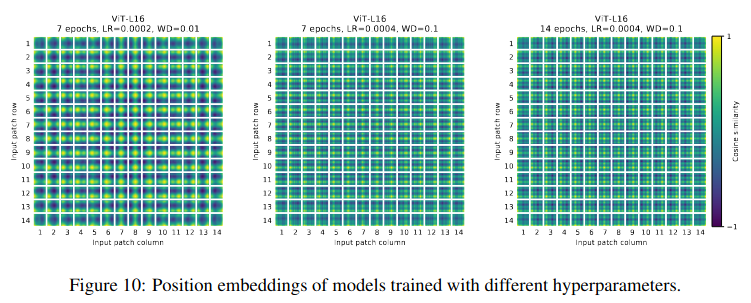1. 对于 $1$-D 的位置编码，作者构造了一个 $196$ 维的位置编码表，表中第一行是一个可学习的位置编码，长度为 $D$
2. 对于 $2$-D 的位置编码，作者分别构造了两个 $196$ 维的位置编码表。一个是行位置编码表，其中每一行长度为 $\frac{D}{2}$；另一个是列位置编码表，其中每一行长度为 $\frac{D}{2}$。求每个 patch 的位置编码时，用其对应的行的位置编码和列的位置编码拼接在一起得到最终的位置编码。

## 5. 实验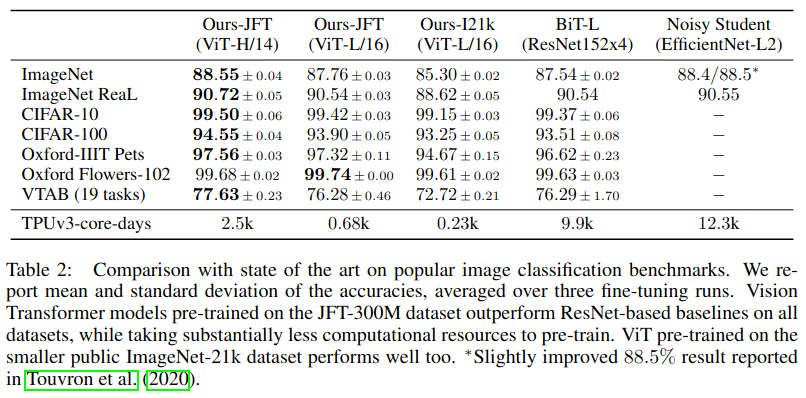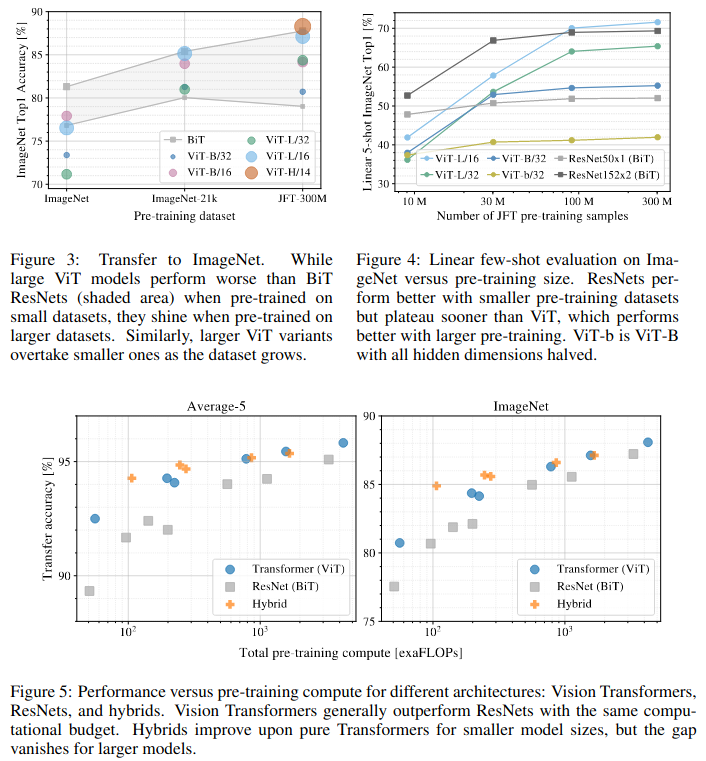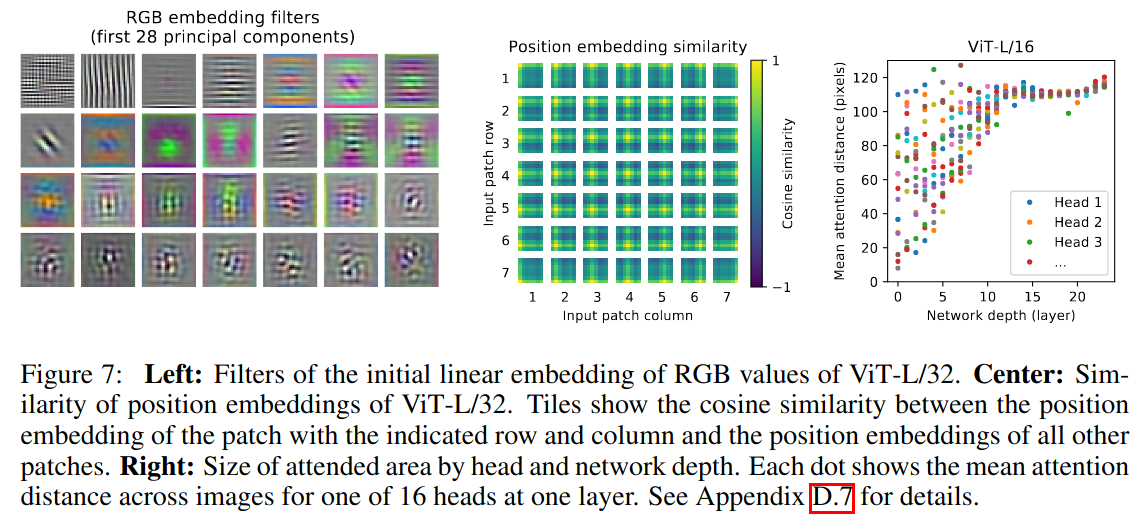• Embedding 层的可视化：可以看到，类似于 CNN 一样，Embedding 层学到了一些 gabor filter ，这些 filter 是可以作为基函数来表示真实的图像 patch；
• 位置编码相似度的可视化：和前面说到的一样，ViT 的确学到了 patch 的位置编码信息；
• 多头注意力距离可视化：可以看到，在比较浅层的网络，多头注意力的确注意到不同距离的图像 patch。

## 附录

1. Dosovitskiy, A., Beyer, L., Kolesnikov, A., Weissenborn, D., Zhai, X., Unterthiner, T., ... & Houlsby, N. (2020, September). An Image is Worth 16x16 Words: Transformers for Image Recognition at Scale. In International Conference on Learning Representations.
2. Xiaolong Wang, Ross Girshick, Abhinav Gupta, and Kaiming He. Non-local neural networks. In CVPR, 2018